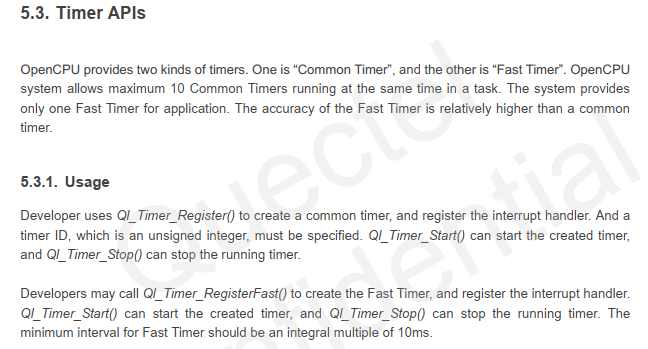# How to use fast timer with MC60 opencpu solution

Hi there,

I want to use fast timer with MC60 opencpu. I don’t have any problem with stack timer. I had used stack stack timer without any problem at all. I am facing issue with fast timer.

If I am using this ret=RIL_GPS_Read(“ALL”,rdBuff); in timer handler it is restarting the module after first run.
However, it is not restarting if I remove ret=RIL_GPS_Read(“ALL”,rdBuff); from timer handler.

Interval is 10 seconds

``````//register  a timer
ret = Ql_Timer_RegisterFast(GP_timer, Timer_handler, &m_param1);
if(ret <0)
{
APP_DEBUG("\r\n<--failed!!, Ql_Timer_Register: timer(%d) fail ,ret = %d -->\r\n",GP_timer,ret);
}
APP_DEBUG("\r\n<--Register: timerId=%d, param = %d,ret = %d -->\r\n", GP_timer ,m_param1,ret);

//start a timer,repeat=true;
ret = Ql_Timer_Start(GP_timer,GPT_Interval,TRUE);
if(ret < 0)
{
APP_DEBUG("\r\n<--failed!! GP timer Ql_Timer_Start ret=%d-->\r\n",ret);
}
APP_DEBUG("\r\n<--GP timer Ql_Timer_Start(ID=%d,Interval=%d,) ret=%d-->\r\n",GP_timer,GPT_Interval,ret);
``````

Timer handler callback:

``````if(GP_timer == timerId)
{
s32 ret=-1;
u8 rd_buff;
APP_DEBUG("<-- GP-Timer_handler, param:%d -->\r\n", *((s32*)param));
//not repeat
//        Ql_sprintf(tx_buffer,"Data ID = %d ", *((s32*)param));
//        GPRS_SendData(tx_buffer);
}
``````

Looking forward how can we run fast timer in free running mode.
Any help will be appreciated. Thank youDear dev

What is the version of SDK you are using?

You are in the timer callback function “ret=RIL_GPS_Read(” ALL ",rdBuff);"Will it restart after reading the data?"Ret = RIL_GPS_Read(“RMC”,rd_buff);"Will it be restarted?

Can we test this out by making “rd_buff” a little bit smaller let’s see, not 1000 bytes, just 200 bytes and try it out.